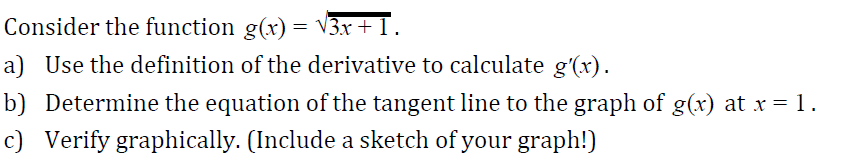Consider the function g(x)- V3ra) Use the definition of the derivative to calculate g'(r)b) Determine the equation of the tangent line to the graph of g(x) at x-1c) Verify graphically. (Include a sketch of your graph!)

Questionhelp_outlineImage TranscriptioncloseConsider the function g(x)- V3r a) Use the definition of the derivative to calculate g'(r) b) Determine the equation of the tangent line to the graph of g(x) at x-1 c) Verify graphically. (Include a sketch of your graph!) fullscreen
Step 1

We have to find derivative of g(x) using definition of derivative ,where g(x) is given by:

Step 2

In this question we will use following rules to find derivative and tangent line.

Rules are given by

Step 3

PART-1:With the help of definition of derivative we have fo...

Want to see the full answer?

See Solution

Want to see this answer and more?

Our solutions are written by experts, many with advanced degrees, and available 24/7

See Solution
Tagged in

Derivative